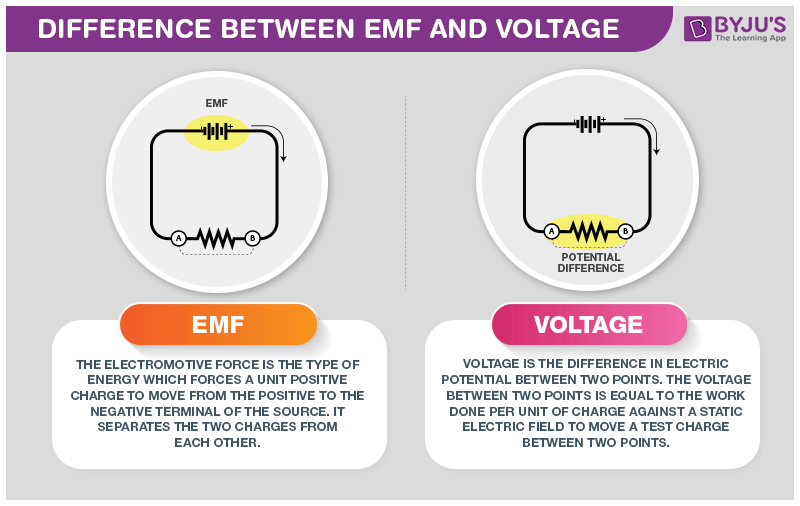# Difference Between EMF and Voltage

In order to know the difference between emf and voltage, we should be aware that EMF stands for electromotive force and is referred to as the voltage present at the ends of the source in the absence of current. And when we close the circuit for letting the electric current flow, then, there is a presence of voltage at the ends of the source.## Difference Between EMF and Voltage

The voltage found is very small compared to emf and it is the result of the internal resistance of the source itself which is leading the drop in the voltage.

EMF is electrically charged particles as a result of the separation of electrons from the atoms by consuming some types of energy such as chemical, mechanical, or light.

EMF= A/Q (where A= amount of work performed and Q= amount of electricity)

Voltage is the difference of the electrical states on the poles. In a closed electrical circuit, electrons move from the negative half to the positive half. Electrical voltage is referred to the work performed by the electric force in moving the charge from one point of the field to another

Difference Between EMF and Voltage
EMF Voltage
It denotes the voltage produced inside the electric source. It is the difference potential difference between two points
External forces work in moving a charge from one pole to another pole inside a source It is the work in moving a charge from one pole to another through a wire
E = I * (R + r) V = I * R
It follows the coulomb force operation It follows a non-coulomb force operation
It measured with EMF meter It is measured with a voltmeter
It is always greater than the voltage It is always lesser than EMF
Its intensity is constant Its intensity is not constant
EMF is caused in a gravitational, electric or magnetic field Voltage is caused only in the electric field

These were some difference between EMF and Voltage. If you wish to find out more, download BYJU’S The Learning App.

RELATED ARTICLES:

Test your knowledge on EMF and voltage differences